# Investment

## Definitions

### Words

"offers", i.e. what people are willing to sell
bids
difference between the best bid and best ask
basically means "buy / sell X amount at the best possible price, right now", which means that if you're not careful, you might end up buying more than what's available at the price you want and thus buying from the next level (asks)
you specify the price and quantity you're willing to buy or sell at

Dividends is a sum of money paid regularly (typically annually) by a company to its shareholders out of its profis (or reserves).

### Alpha

Alpha is a measure of the active return on an investment, the performance of that investment compared with a suitable market index.

It takes the volatility (price risk) of a security or fund portfolio and compares its risk-adjusted performance to a benchmark index. The excess return of the investment relative to the return of the benchmark index is its alpha.

An alpha of 1% means the investment's return on investment (ROI) over a selected period of time was 1% better than the market during the same period.

### Beta

Beta (coefficient) is a measure of the volatility, or systematic risk, of a security or a portfolio in comparison to the market as a whole.

Beta is calculated using regression analysis, and you can think of it as the tendency of an investment's return to respond to swings in the market. By definition, the market has a beta of 1.0. Individual security and portfolio values are measured according to how they deviate from the market.

### Sharpe ratio

The Sharpe ratio is defined as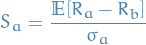where

•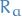is the asset return
•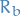is the risk-free rate
•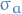is the std. dev. of the asset

### Markets

Equity market is the market in which shares are issued and traded, either through exchanges or over-the-counter markets, also known as the stock market.

Credit market refers to the market through which companies and governments issue debt to investors, such as investment-grade bonds, junk bonds and short-term commercial paper.

Sometimes called the debt market, the credit market also includes:

• debt offerings, e.g. notes
• securitized obligations, including
• mortgage pools
• collateralized debt obligations (CDOs)
• dwarfs
• credit default swaps (CDS)

## Capital Asset Pricing Model (CAPM)

Capital asset pricing model (CAPM) is a model used to determine a theoretically appropriate required rate of return of an asset, to make decisions about adding assets to a well-diversified portfolio.

In CAPM, for individual securities, we make use of the security characteristic line (SCL) , which is the regression line, modeling the performance of a particular security or portfolio against that of the market portfolio at every point in time: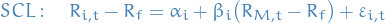where

•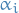is called the asset's alpha (abnormal return)
•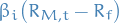is a nondiversifiable or systematic risk
•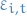is the non-systematic or diversifiable, non-market or idiosyncratic risk
•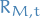is a market risk, or rather the return of the market portfolio
•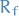is a risk-free rate (e.g. interest rates from keeping the money in the bank)

Minimizing the RSS (i.e. obtaining the MLE), we get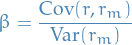where we've let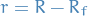and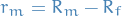, which is in coherence with the definition of beta as "relative variance / risk wrt. market".

## Volatility

Simply the variance of the financial instrument.

## Risk

• Reflects the chance that the actual return on an investment varies from the expected return (i.e. mean)
• There a multiple measures of risk:
• Variance / std. dev.

## Sharpe Ratio

### Overview

• Measure for computing risk-adjusted return

## Risk-free return

• Theoretical rate of return of an investment with zero risk
• Considering risk is a variance metric, I'm assuming the risk-free return is then simply the expected or average return

## DoM - Depth of Market

Basically about observing the order-book, i.e. the volumes of the bids and sells (both those "scheduled" and those being performed).

## Average Portfolio Allocation to Equities

This is heavily based on this article. It's about how one should look into using Average Portfolio Allocation to Equities as a predictor of future stock market returns. The definition argued for is the following: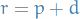1

where

•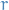is the total return 1
•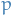is the price return 2
•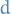is the dividends return 3

Furthermore, we can write the price return term as follows: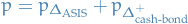2

where:

•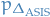is the price return from change in Aggregate Investor Allocation of Stocks 4
•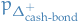is the price return from increase in Cash-Bond supply 5, the value of which assumes Aggregeate Investor Allocation of Stocks stays constant

Why this formula? It's all explained very well in the article, but to summarize:

• Assuming you can diversify your investments; if the funds spent on stocks goes up, i.e. aggregate investor allocation of stocks, your return goes up.
• If the Cash-Bond supply increases:
• if the loaner takes a loan from the bank → money supply expands → investors have more money → (assuming stock allocation for the investor stays constant) investor invests more money into stocks
• if the loaner takes a loan directly from the investor → investor holds the bond → investor now has more bonds than the allocated ratio → need to increase investment in stocks to get back to his/her set stock allocation
• Note: the average bond trades close to par, so, in aggregate, the value of the liabilies approximates the total market value of the bonds.

## Footnotes:

1

Total return is the rate of return of an investment portfolio, including capital appreciation, interests and divideds.

2

Price return is the rate of return on an investment portfolio, where the return measure takes into account only the capital appreciation of the portfolio, while income generated by the asets in the portfolio, in the form of interest and dividends, is ignored. This contrasts with the total return, which does take into account the income generated in the portfolio.

3

Dividends is a sum of money paid regularly (typically annually) by a company to its shareholders out of its profis (or reserves).

4

Aggregate Investor Allocation of Stocks is the summed up funds to be allocated in the stock market

5

Cash-Bond supply is the supply of cash and bonds, i.e. money NOT allocated to for investments in stocks.